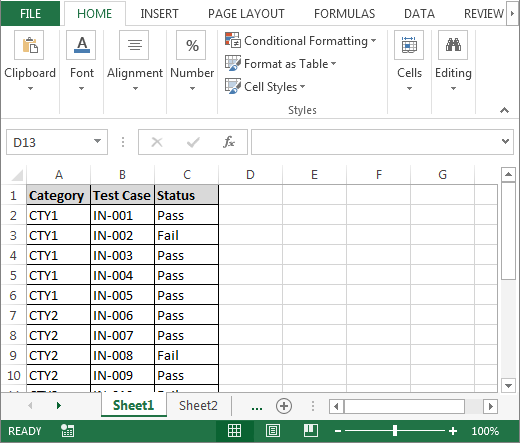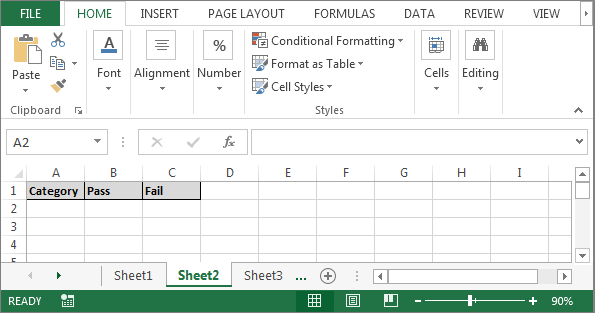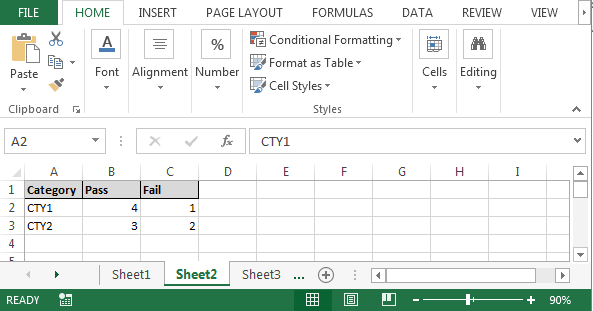# Count Cells through Excel VBA

In this article, we are going to learn how to count and display total through VBA in Microsoft Excel.

Let’s understand with a simple exercise, how and where we can count and display totals through VBA in Microsoft Excel.

We have data in Sheet 1 in which column A contains category, column B contains Competitor Id and column C contains status.

Now, we want to retrieve a report in Sheet2 in the same workbook that contains the data of passed and failed candidates, categorically.• Press the key Alt+F11 to open the VBE page to write the macro.
• Then go to insert tab and insert a module.
• Write below mentioned code in the page.

```Sub CountStatus()
Dim Lastrow As Long, Countpass1 As Long, countfail1 As Long
Dim erow As Long, Countpass2 As Long, CountFail2 As Long

Lastrow = Sheet1.Cells(Rows.Count, 1).End(xlUp).Row
erow = Sheet2.Cells(Rows.Count, 1).End(xlUp).Offset(1, 0).Row

Countpass1 = 0
countfail1 = 0
Countpass2 = 0
CountFail2 = 0

For i = 2 To Lastrow
If Sheet1.Cells(i, 1) = "CTY1" And Sheet1.Cells(i, 3) = "Pass" Then
Countpass1 = Countpass1 + 1
ElseIf Sheet1.Cells(i, 1) = "CTY1" And Sheet1.Cells(i, 3) = "Fail" Then
countfail1 = countfail1 + 1
ElseIf Sheet1.Cells(i, 1) = "CTY2" And Sheet1.Cells(i, 3) = "Pass" Then
Countpass2 = Countpass2 + 1
ElseIf Sheet1.Cells(i, 1) = "CTY2" And Sheet1.Cells(i, 3) = "Fail" Then
CountFail2 = CountFail2 + 1
End If
Next i

'Msgbox "Pass count of CTY1," & " " & Countpass1 & " " & "Fail Count of CTY1," & " " & countfail1 & vbCrLf & "Pass count of CTY2," & " " & Countpass2 & " " & "Fail Count of CTY2," & " " & CountFail2
Sheet2.Range("A2:C500").Clear
Sheet2.Cells(erow, 1) = "CTY1"
Sheet2.Cells(erow, 2) = Countpass1
Sheet2.Cells(erow, 3) = countfail1
erow = erow + 1
Sheet2.Cells(erow, 1) = "CTY2"
Sheet2.Cells(erow, 2) = Countpass2
Sheet2.Cells(erow, 3) = CountFail2

End Sub
```

• To run the macro, press the key F5.
• All details will get updated in Sheet2 as per the requirement.This is the way to count and display totals through VBA in Microsoft Excel.